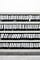# Keeping Pandas DataFrames clean when importing JSONPhoto by Samuel Zeller on Unsplash
`from pandas.io.json import json_normalizedf = json_normalize(data)`

# Ugly: Keeping imported columns

`from pandas.io.json import json_normalizedf = json_normalize(data)df['company_id'] = df['companyId']df['location'] = df['properties.city.value']df['name'] = df['properties.name.value']df['domain'] = df['properties.website.value']//... .apply(), .as_type(int), whatever...`

# Ugly: Dropping columns manually

`from pandas.io.json import json_normalizedf = json_normalize(data)// make temporary columnsdf.columns = ['temp_' + c for c in df.columns]// pre-processing, basic calculations, etc.df['company_id'] = df['temp_companyId']df['location'] = df['temp_properties.city.value']df['name'] = df['temp_properties.name.value']df['domain'] = df['temp_properties.website.value']//... .apply(), .as_type(int), whatever...`
`df.drop([c for c in df.columns if c.startswith('temp_')], axis=1, inplace=True)// ordf = df[[c for c in df.columns if not c.startswith('temp_')]]`

# Clean and easy: using a Context Manager

`with DataFrameFromDict(companies) as df:    // imported dict now in df, same result as json_normalize    df['company_id'] = df['companyId']    df['location'] = df['properties.city.value']    df['name'] = df['properties.name.value']    df['domain'] = df['properties.website.value']// after context exits, df contains company_id, location, name, and domain// but no more temporary columnsprint(df)`

# How it works

`class DataFrameFromDict(object):    """    Temporarily imports data frame columns and deletes them afterwards.    """    def __init__(self, data):        self.df = json_normalize(data)        self.columns = list(self.df.columns.values)    def __enter__(self):        return self.df    def __exit__(self, exc_type, exc_val, exc_tb):        self.df.drop([c for c in self.columns], axis=1, inplace=True)`

Techie, Hacker, Millenial, founding a VC, @_firstmomentum. #venturecapital #deeptech #socialnetworkanalysis #automation #crawlers #ML #HR

## More from Karl Lorey

Techie, Hacker, Millenial, founding a VC, @_firstmomentum. #venturecapital #deeptech #socialnetworkanalysis #automation #crawlers #ML #HR